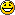¡Esta es una revisión vieja del documento!

Tabla de Contenidos

# Density of a body

## What´s density? What´s it used?

The density is a magnitude used by the physics and chemistry to determine the amoun of mass contained in a given volume.

There are two types of densities:

• ABSOLUT DENSITY: the density, which measure the mass per unit volume and is usually known by density.

Therefore, to calculate the density of a body you have to consider the following formula:or that´s the samewhere –mass is the number of particles that make up the substance or body. The mass is measured in grams.
(What´s the difference between mass and weight of a body?)
–and the volume is the total number of scattered molecules.
The volume is in cubic units ().

• RELATIVE DENSITY: is the density that compares the density of a substance with the density of water.

Is calculated using the followingo formula:or that´s the sameThe density of water isor that´s the same:?:Observes these two buckets, which belives it has greater density?

Because we know that density is the amount of mass contained in a given volume, it is easy to see that as the buckets are equal (have the same volume) and B in the cube is more number of particles (ie, higher mass), the density of the bucket B is greater than the density of the cube A.:?:Observe the following photograph of the globular cluster M13 or Hercules globular cluster:We take two regions of the cluster, A and B.
For the same volume (square of 12*12 light years), we see that the area A accommodates smaller number of stars that the area B .

Which region has the lowest density?
A volume equal to the two areas, area B contains the largest number of stars in zone A, therefore the density of A is less than the density of B.In nature, the matter can be presented in three different states: solid, liquid or gaseous.

Let´s go to look some examples of densities of various elements and substances most common and known to us.

Some solids are:

 Sodium Diamond Silicon dioxide Cesium chloride 0.97 3.5 2.6 3.99

Some liquids are:

 Water Ethanol Essential Oil Petrol 1 0.79 0.92 0.71
 Ozone Carbon Dioxide Air Ammonia 2.14 1.6 1.18 0.73

The data given in this section of gases, are data in normal pressure and temperature, ie:

pressure = 1 atmosphere           temperature = 0º = 273 K

##### Example

A ball of radius 51.6 cm is composed of 40% iron and the rest is aluminium.

a)What is the area and volume of the ball?
(What are the area and volume of a spherical body?)

b)What is the weight of the ball?
(What is the weight of a body?)

a) The area of any sphere is:As the radius of the ball is R=15.6 cm, the area is:= 3058 square centimeters.

The volume of a sphere is equal to:As the radius of the ball is R=15.6 cm, the volume turns out to be:= 15902.4 square centimeters.

b) To calculate the weight of the ball we need to know the densities of the materials that comprise it.
The density of iron is:The density of aluminium is:For the definition of density:. Then the mass of the ball is:.mass = (7.84×0.4 + 2.7×0.6)*15902´4 = 75759 grams

Therefore, for a body in the earth´s surface, where g = 9.8, the weight is:

weight = 75´759 x 9.8 = 742 Newton approximately.

• BUOYANCY

What is buoyancy?

The density of a body is related to the buoyancy: a substance will float on the other if their density is lower.

:?:What happens when we launch a paper airplane to water?The density of the paper is 0.77 g/cm³ and the density of water is 1 g/cm³, therefore, at the begining the paper fleet becouse the density of the sheet is lower than the density of water.After a short period of time, just need to soak the sheet and become a grater density (particles bind water and cellulose), the blade falls to the bottom because the takes a higher density than the water.